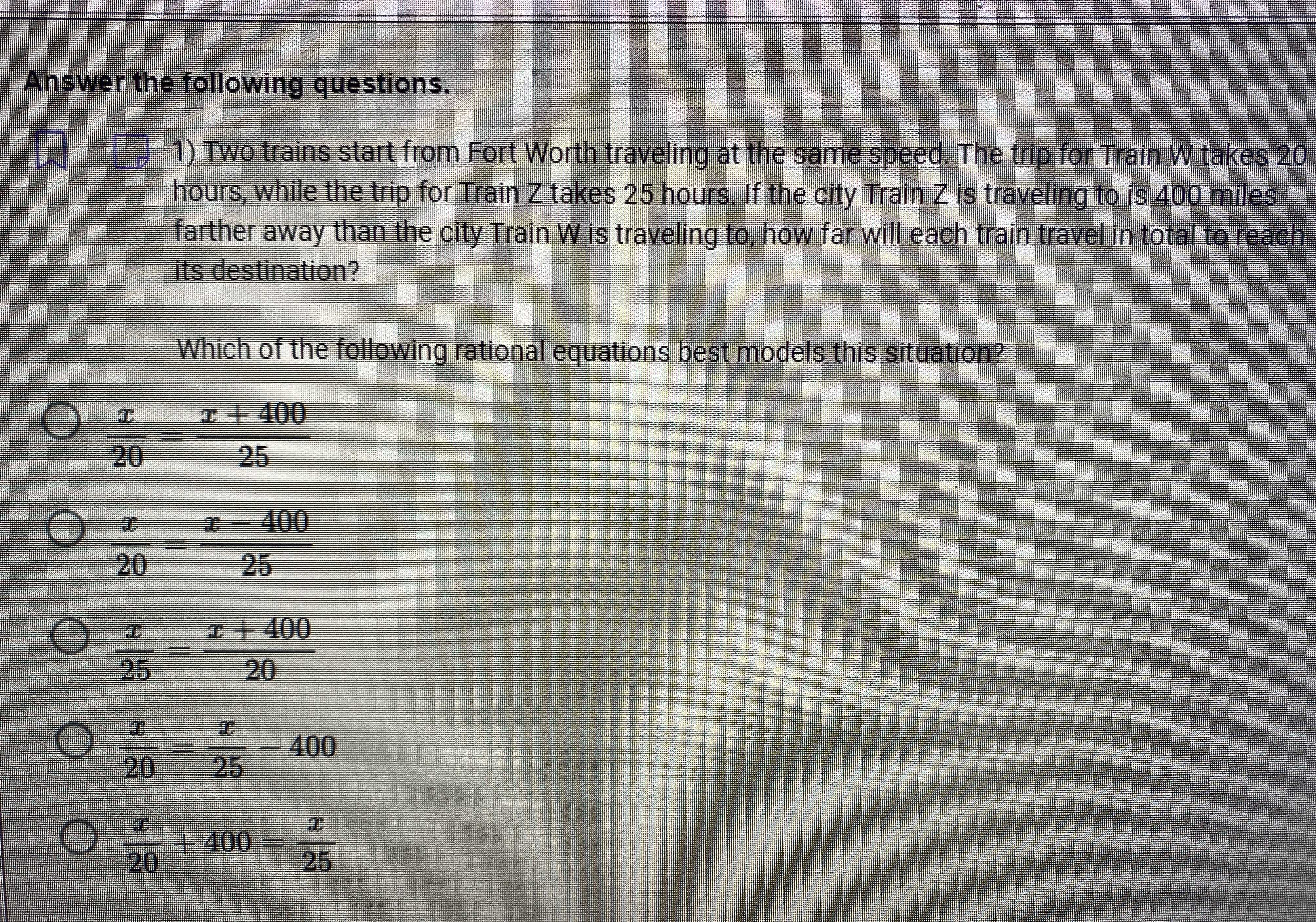### ¿Todavía tienes preguntas de matemáticas?

Pregunte a nuestros tutores expertos
Algebra
Pregunta1) Two trains start from Fort Worth traveling at the same speed. The trip for Train $$W$$ takes $$20$$ hours, while the trip for Train $$Z$$ takes $$25$$ hours. If the city Train $$Z$$ is traveling to is $$400$$ miles farther away than the city Train $$W$$ is traveling to, how far will each train travel in total to reach its destination?

Which of the following rational equations best models this situation?

$$\frac { x } { 20 } = \frac { x + 400 } { 25 }$$

$$\frac { x } { 20 } = \frac { x - 400 } { 25 }$$

$$\frac { x } { 25 } = \frac { x + 400 } { 20 }$$

$$\frac { x } { 20 } = \frac { x } { 25 } - 400$$

$$\frac { x } { 20 } + 400 = \frac { x } { 25 }$$

$$\frac { x } { 20 } = \frac { x + 400 } { 25 }$$